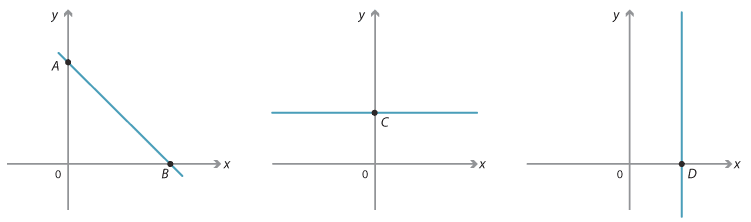### x- and y- intercepts

The x-intercept of a line is the x-value of the point at which the line crosses the x-axis.

The y-intercept of a line is the y-value of the point at which the line cuts the y-axis.Detailed description

In the diagram to the left the y-intercept is at A and the x-intercept at B.
The second diagram shows a line parallel to the x-axis and it has a y-intercept at C.
The third diagram shows a line parallel to the y-axis and it has an x-intercept at D.